• 傅里叶级数、一维傅里叶变换到二维傅里叶变换数理推导 参考资料： 如何理解傅里叶级数公式 从傅里叶级数到傅里叶变换 高维傅里叶变换的推导 IDL实现傅里叶变换 傅里叶级数 一维傅里叶变换 二维傅里叶变换 ...
傅里叶级数、一维傅里叶变换到二维傅里叶变换数理推导
参考资料： 如何理解傅里叶级数公式 二重傅里叶级数 从傅里叶级数到傅里叶变换 高维傅里叶变换的推导 连续傅里叶变换和离散傅里叶变换 二维离散傅里叶变换 IDL实现傅里叶变换
想要用傅里叶变换的思维处理一个时序数据，将时序信息转换为参数信息，实现降维的目的。大致了解了一下傅里叶级数，如何从傅里叶级数转到傅里叶变换以及一维傅里叶变换和二维傅里叶变换的过渡，傅里叶变换的求解，快速傅里叶变换等知识。 傅里叶变换是一种从时域信息转为频域信息的手段，可以理解为从不同的角度来观察一个事物，例如总是被用来举例的听到的钢琴曲和乐谱，就是一个是时域一个频域，但是无论是时间维度听到的钢琴声还是频域维度看到的乐谱，他俩实质上描述的东西是一样的。
傅里叶级数
首先是傅里叶级数的概念以及这个概念被提出来的初衷。 最早，数学家发现某些周期函数可以由三角函数的和来表示，后来又有人猜测任意周期函数都可以写为三角函数之和。只需要三角函数之和函数满足以下几点：1）有常数项；2）同时包含奇函数和偶函数来组合出任意函数；3）周期与原函数周期相同，为T;4)可以调整振幅来逼近原函数（也就是系数项）。 构造出来的三角函数之和函数大致如下：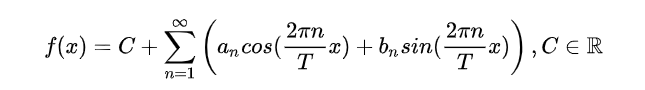接下来的重点是如何来确定参数：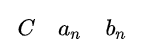在确定参量的时候，引入欧拉公式：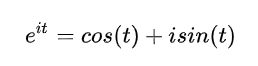最终得到的系数表达式为：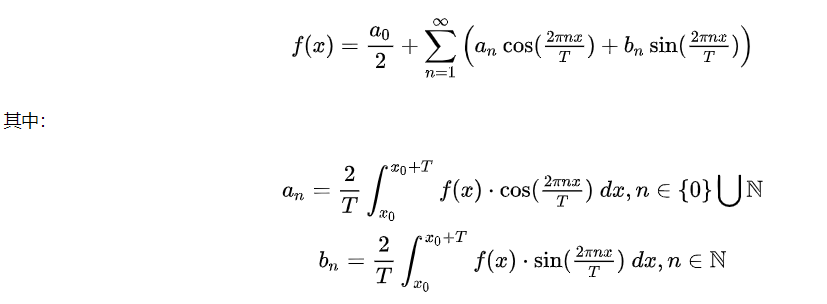具体的函数构造以及求解原理可以参照博客如何理解傅里叶级数公式。
傅里叶变换
傅立叶级数是基于周期函数的，如果我们把周期推广到无穷，那么也就变为了非周期函数，这就是傅立叶变换。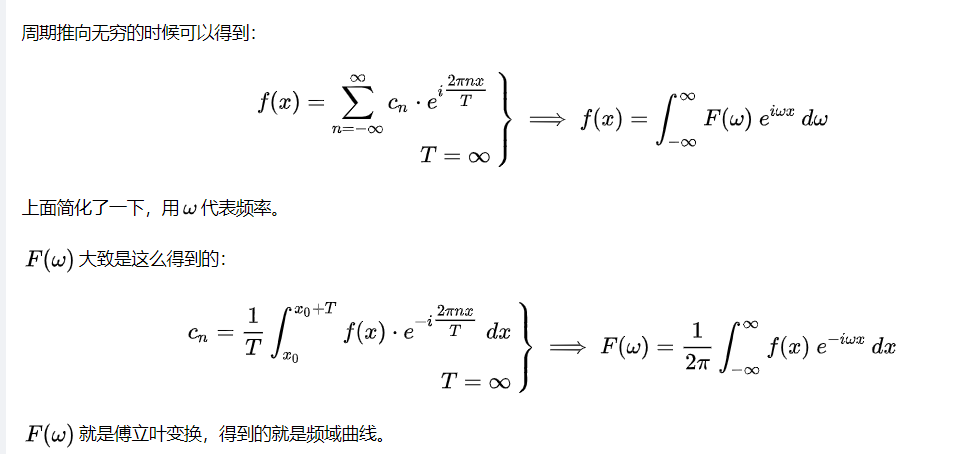一维傅里叶变换到二维傅里叶变换
对于傅里叶变换从一维到二维的扩展，主要参照知乎的一个回答 类比于一维傅里叶变换将一个函数分解成若干个一维的简单函数（正弦波）之和，二维傅里叶变换将一个图像分解成若干个正弦平面波之和。如下图：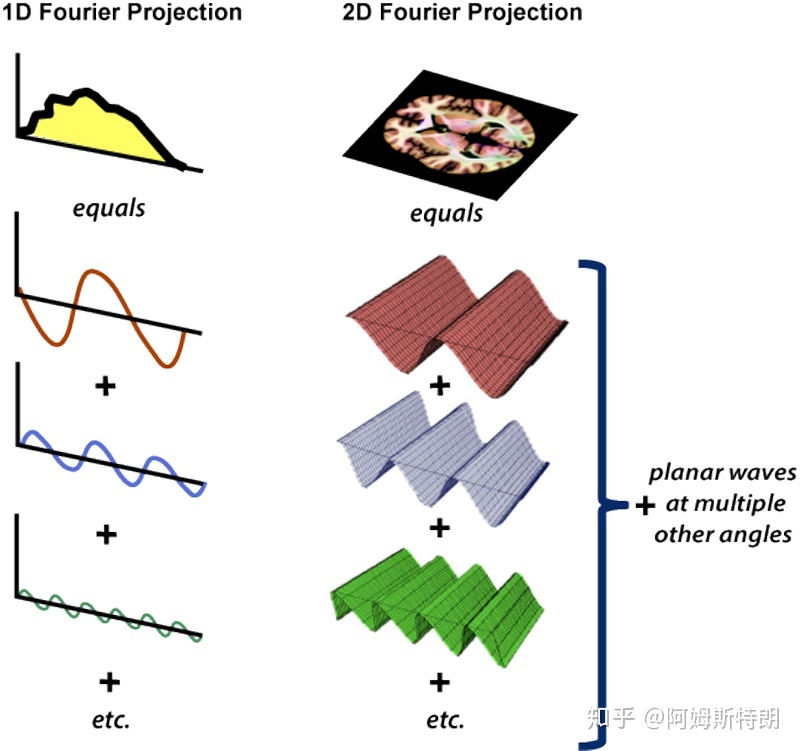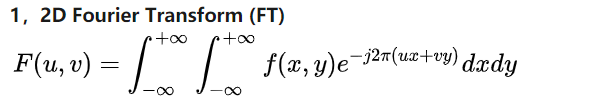二维离散傅里叶变换 二维离散傅里叶变换DFT可分离性的基本思想是DFT可分离为两次一维DFT。因此可以用通过计算两次一维的FFT来得到二维快速傅里叶FFT算法。根据快速傅里叶变换的计算要求，需要图像的行数、列数均满足2的n次方，如果不满足，在计算FFT之前先要对图像补零以满足2的n次。 一个M行N列的二维图像f(x,y)，先按行队列变量y做一次长度为N的一维离散傅里叶变换，再将计算结果按列向对变量x做一次长度为M傅里叶变换就可以得到该图像的傅里叶变换结果，如式所示：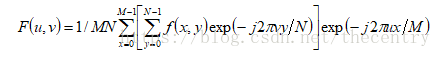将上式分解开来就是如下的两部分，先得到F(x,v),再由F(x,v)得到F(u,v)：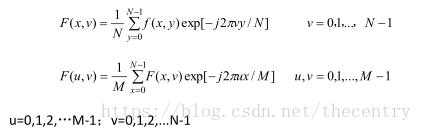每一行由N个点，对每一行的一维N点序列进行离散傅里叶变换得到F(x,u),再对得到F(x,u)按列向对每一列做M点的离散傅里叶变换，就可以得到二维图像f(x,y)的离散傅里叶变换F(u,v).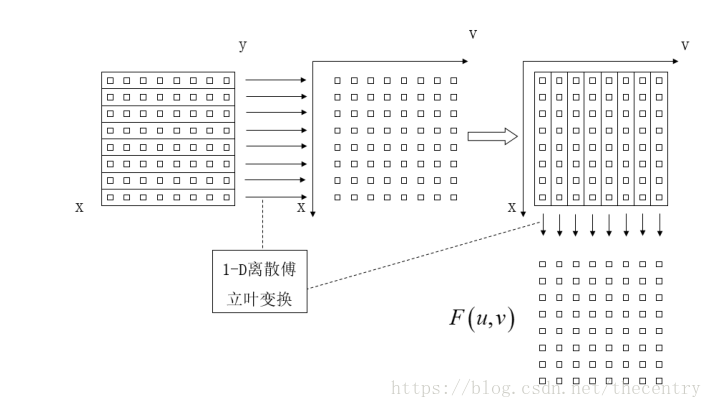同样，做傅里叶逆变换时，先对列向做一维傅里叶逆变换，再对行做一维逆傅里叶变换，如下式所示：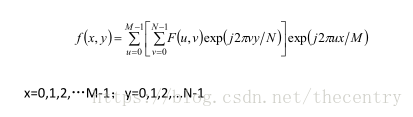展开全文• 看了一个论文说二维傅里叶变换图谱经圆积分计算后可以得到一维傅里叶变换，求大神推导公式或者过程
• 回顾一下一维FT公式： 通俗来讲，一维傅里叶变换是将一个一维的信号分解成若干个复指数波。而由于 ，所以可以将每一个复指数波 都视为是 余弦波+j*正弦波的组合。对于一个正弦波而言，需要三...

点击上方“机器学习与生成对抗网络”，关注"星标"
获取有趣、好玩的前沿干货！
来自 | 知乎  阿姆斯特朗
链接 | https://zhuanlan.zhihu.com/p/110026009
文仅交流，侵删
1.回顾一下一维FT
公式：通俗来讲，一维傅里叶变换是将一个一维的信号分解成若干个复指数波。而由于，所以可以将每一个复指数波都视为是
余弦波+j*正弦波的组合。
对于一个正弦波而言，需要三个参数来确定它：频率,幅度，相位。因此在频域中，一维坐标代表频率，而每个坐标对应的函数值也就是是一个复数，其中它的幅度就是这个频率正弦波的幅度，相位就是。下图右侧展现的只是幅度图，在信号处理中用到更多的也是幅度图。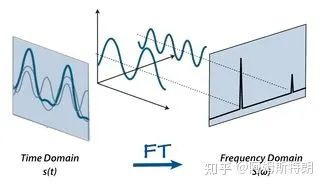一维傅里叶变换就是一个基变换，在时域中，基是一族冲激信号,在频域中；基是，而且这组基是正交基。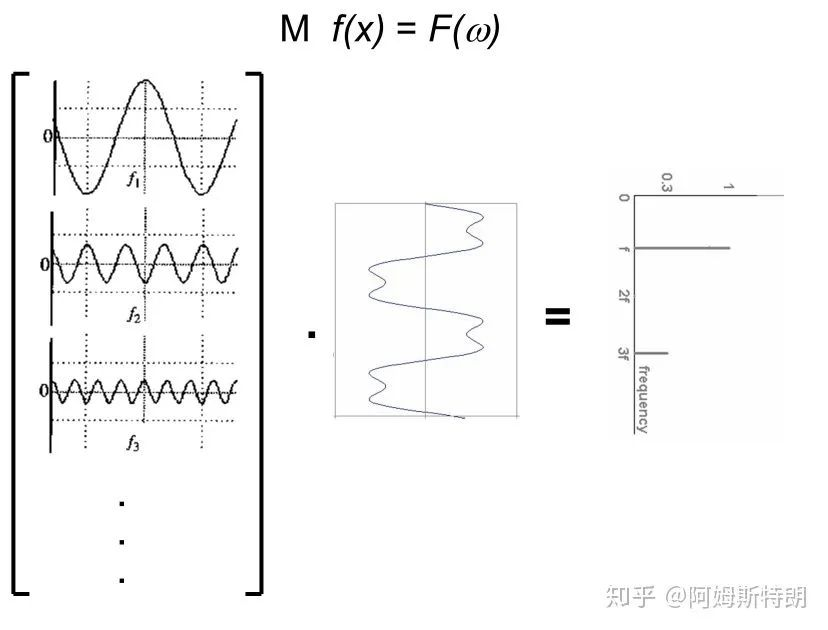F=Mf，基变换示意图
2.类比：从一维到二维
一维信号是一个序列，FT将其分解成若干个一维的简单函数之和。二维的信号可以说是一个图像，类比一维，那二维FT是不是将一个图像分解成若干个简单的图像呢？
确实是这样，
二维FT将一个图像分解成若干个复平面波之和。如下图：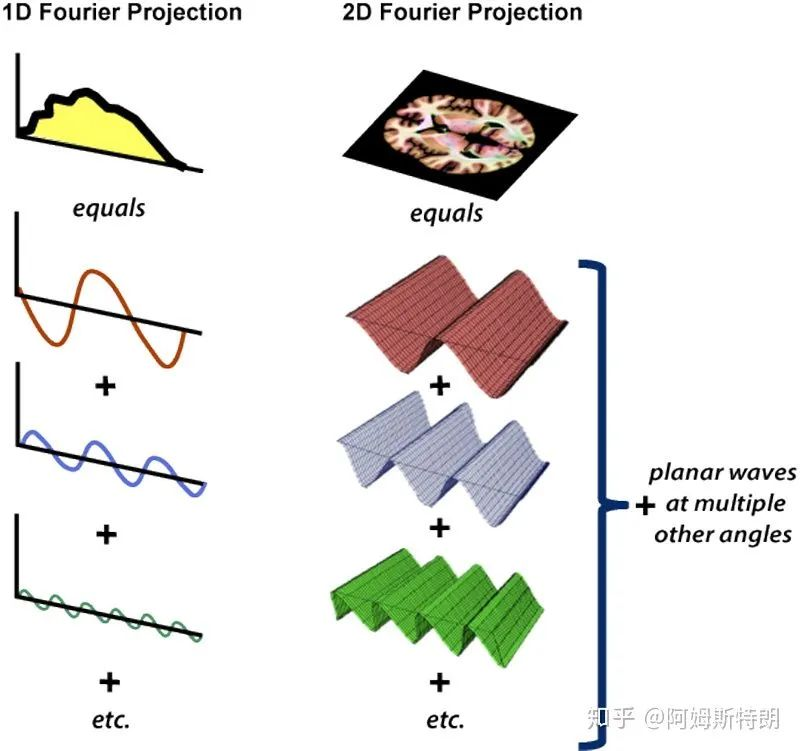二维FT的公式:通过公式，我们可以计算出，
每个平面波在图像中成分是多少。从公式也可以看到，二维傅里叶变换就是将图像与每个不同频率的不同方向的复平面波做内积(先点乘在求和)，也就是一个
求在基上的
投影的过程。(应该知道是b在a上的投影，只不过这里的|a|的值被设为1，所以只有内积)
3.什么是二维频率域K-SPACE
对于正弦平面波，可以这样理解，在一个方向上存在一个正弦函数，在法线方向上将其拉伸。前面说过三个参数可以确定一个一维的正弦波。哪几个参数可以确定一个二维的正弦平面波呢？答案是四个，其中三个和一维的情况一样(频率,幅度，相位)，但是具有相同这些参数的平面波却可以有不同的方向。如下图所示：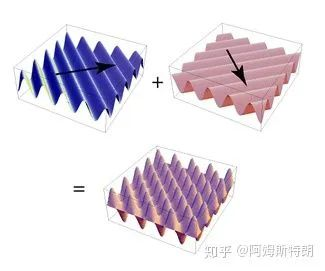两个不同方向的平面波叠加
类比一维中，幅度和相位可以用一个复数表示，它可以作为我们存储的内容。但是还有两个：一个频率一个方向。这时想到向量是有方向的，也是有长度的。所以我们用一个二维的矩阵的来保存分解之后得到的信息。这个矩阵就是K空间。(一般用k来表示空间频率，单位是1/m)
什么意思呢？就是说一个二维矩阵点代表这个平面波的法向量，这个向量的模代表这个平面波的频率，这个点里面保存的内容复数就是此平面波的幅度和相位。下面这个图很好的体现了这一点：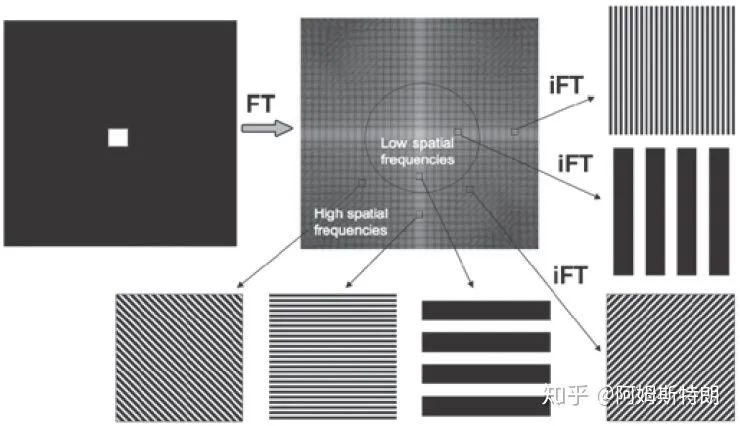也因此K空间的中心对于低频，周围对于高频。如下图，K空间中只有(0，0)处有值，也就是信号都是直流即不存在变化，所以实空间就是一张白纸。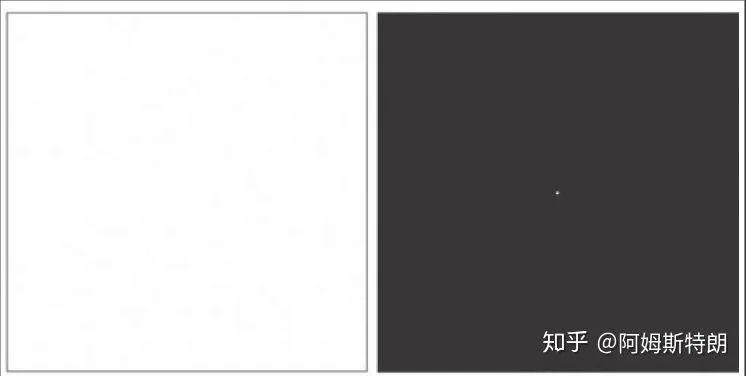再如下面这个图片，
中心低频贡献了图像的主体，周围高频提供图像的细节和边缘。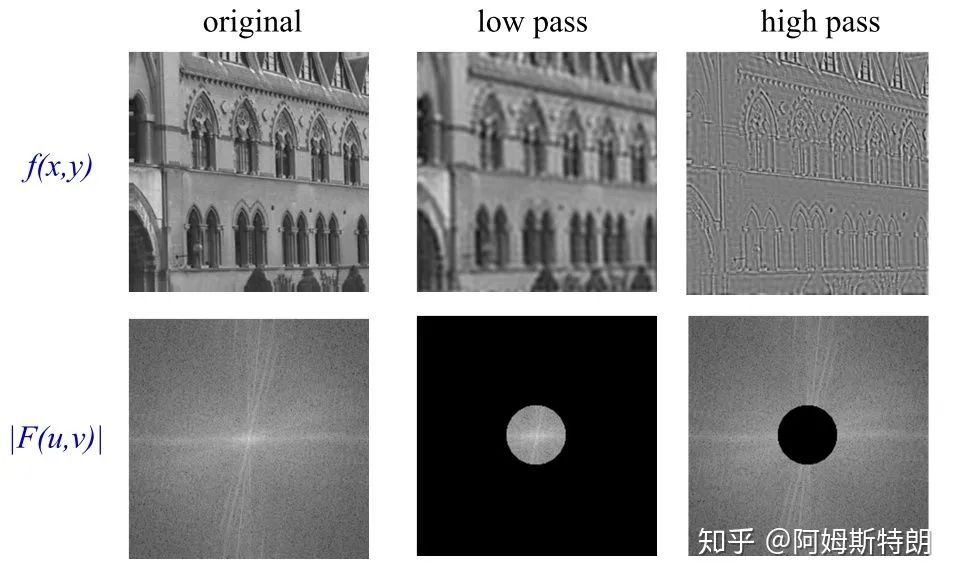因此，k空间的每一个位置存储的数代表了所在位置复平面波在图像中占多少成分，我们就可以用
每个系数*所代表的平面波相加得到原来的图像，也就是下图。
所以k空间和对应图像储存的信息含量是一样的，只不过表现形式不同，或者说基不同。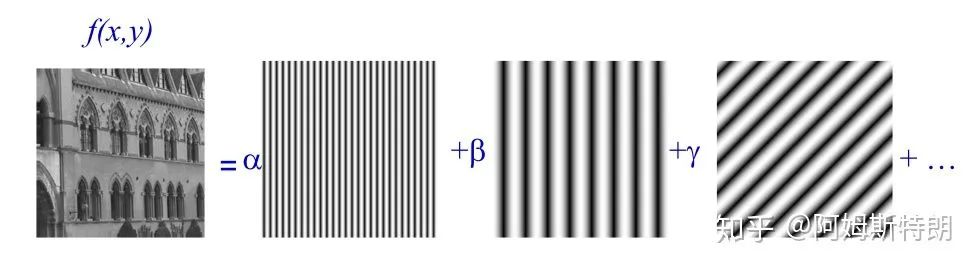4.K空间的一些性质
离散的2D-FT
在数字图像中，数据都是离散的。也就涉及到采样的问题，和一维一样，如果采样率过低，k空间就会混叠。同时在k空间中采样过低，图像也会混叠。
FOV和分辨率在k空间和图像中是相反的关系。也就是：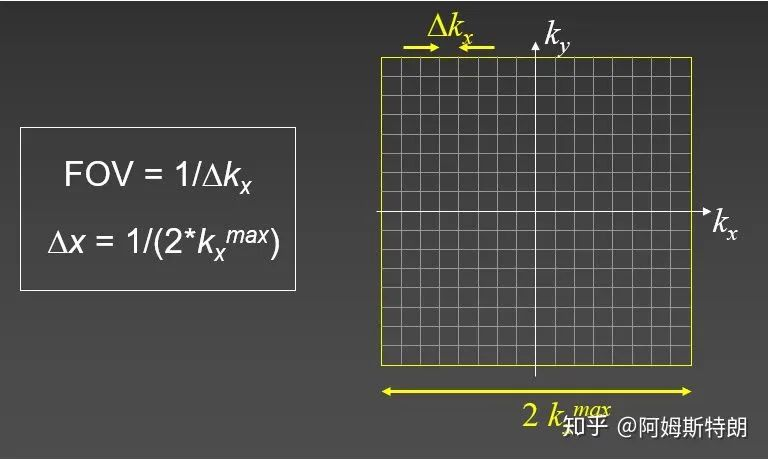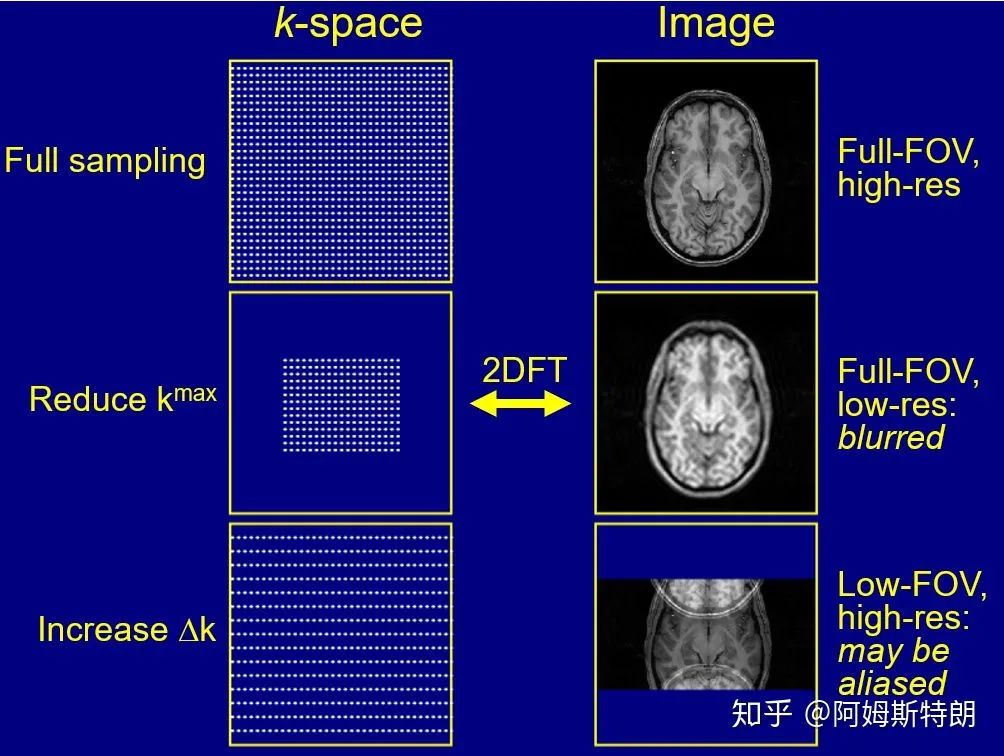减小kmax，使得图像分辨率下降，减小Δk，使得FOV缩小。
旋转不变性
从平面波的角度很容易理解，旋转没有改变平面波的幅度相位，只是将所有的平面波都旋转了一个角度。下面这个图像显示了二维傅里叶变换中，实空间旋转多少，频率空间也会相应旋转多少。这其实是
高维傅里叶变换缩放定理的一种特殊情况。(连续的是可以证明的，离散的涉及插值 ，不一定完全准确)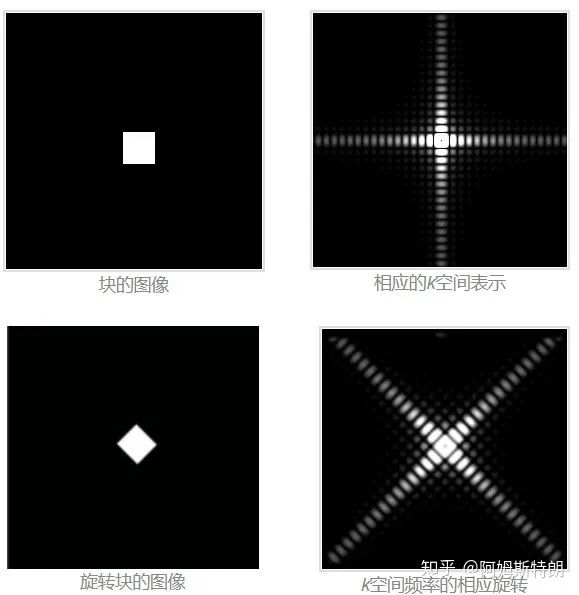5.其他
1.因为matlab中的fft算法都是将0放在第一个的，所有写matlab时一定要将k空间fftshift一下使得零频回到k空间中心。
2.简单的应用k空间进行去噪例子。通过去掉明显的k空间的异常峰，可以去除图像中有规律变化的噪声或者伪影。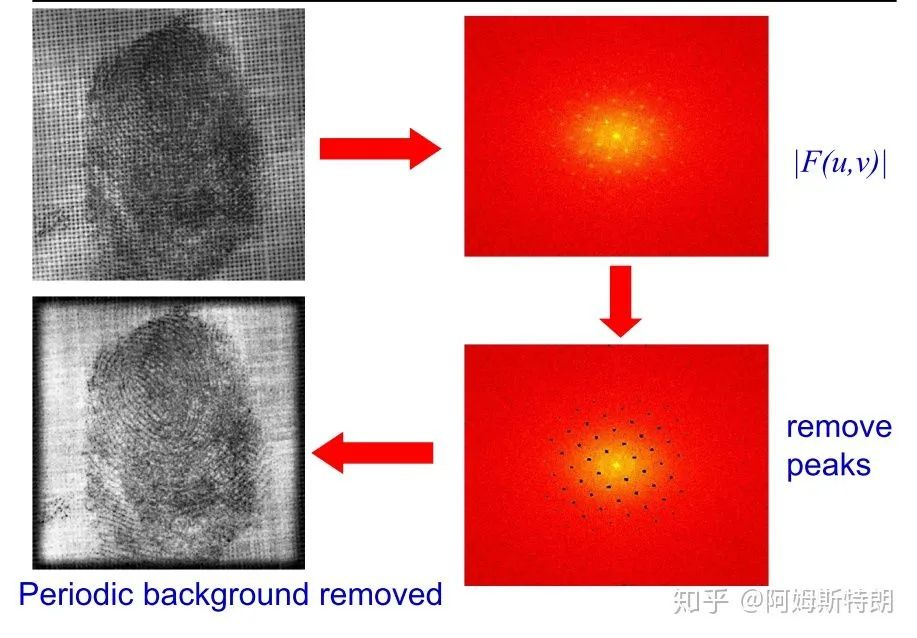指纹去噪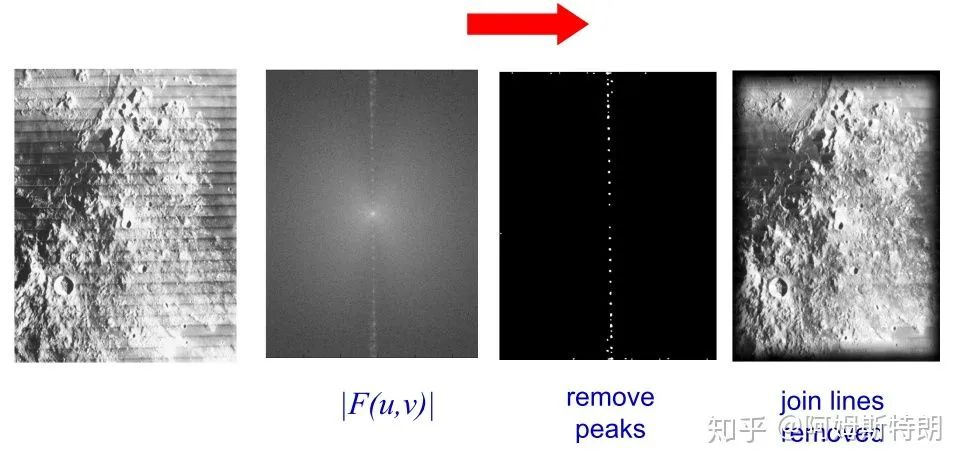天体表面去噪
6、评论及解答
1、始终想不通为啥各个波的叠加能反应出某一个像素点的信号大小
你把它理解向量分解就懂了，平面波就是基，k空间里的数就是基的系数。你得到系数(k空间)的时候就是在投影，变为原向量(图像)就是叠加。只不过这里一个图像是一个向量而已。
复平面波叠加时，先经过放大(幅度)在经过移位(相位)，相位信息里保留了很多位置信息，可以查看我的另一个回答：
为什么用图像二维傅里叶变换的相位谱进行反变换，能够大致得到原图的形状，而幅度谱则不行呢？
2、傅里叶变换后图像是关于频率矩形中心对称的，那么对称的4个平面三角波不就是注定了幅值和相角，频率是相等的。那么这样不是说明了任何波都是包含了4个这样对称的三角波，由它们组成？
是中心共轭对称，而没有左右上下的对称。而且只有实数图像的k空间才有这种特点，这是为了将复平面波中的虚部抵消掉，只留下实数部分。复数图像的k空间没有共轭对称的特点。下面是k空间的一部分数据，中心点为(101，101)。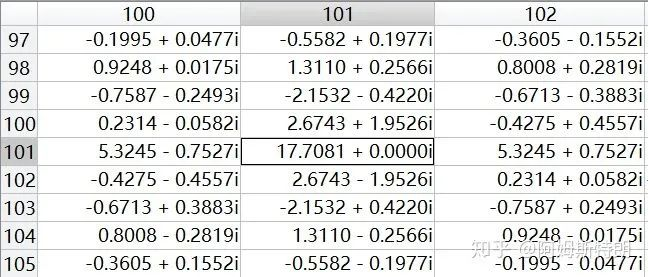参考：
 http://mriquestions.com
 A. Zisserman's lecture in B14 Image Analysis
链接：http://www.robots.ox.ac.uk/~az/lectures/ia/lect2.pdf
(这个slides真的不错，大家可以下载看看，里面也有其他的内容)

展开全文• 二维傅里叶变换的矩阵表示二维傅里叶变换公式二维傅里叶矩阵公式表达1. F(v,u)F(v,u)F(v,u) 为 R1×1R^{1\times1}R1×1 时2. F(v,u)F(v,u)F(v,u) 为 RNv×MuR^{N_v\times M_u}RNv​×Mu​ 时 说明： 做完了图像处理...


二维傅里叶变换的矩阵表示
二维傅里叶变换公式二维傅里叶矩阵公式表达1.

F

(

v

,

u

)

F(v,u)

为

R

1

×

1

R^{1\times1}

时2.

F

(

v

,

u

)

F(v,u)

为

R

N

v

×

M

u

R^{N_v\times M_u}

时

说明： 做完了图像处理的实验报告，老师要求自己编二维傅里叶变换的算法，故不能用
Matlab 内置的
fft2 函数。循环求解会消耗大量的时间，考虑到
Matlab 的优势就在于矩阵运算，故有如下思考。

（如有笔误，欢迎指正；转载请通知作者并注明来源，谢谢亲噢~）

二维傅里叶变换公式
离散时间变量二维傅里叶变换(DFT)的原始公式为：

F

(

u

,

v

)

=

∑

x

=

0

M

−

1

∑

y

=

0

N

−

1

f

(

x

,

y

)

e

−

j

2

π

(

x

M

u

+

y

N

v

)

F(u,v) = \sum_{x=0}^{M-1}\sum_{y=0}^{N-1}f(x,y)e^{-j2\pi(\frac{x}{M}u+\frac{y}{N}v)}

傅里叶变换具有可分性，所以我们有

F

(

u

,

v

)

=

∑

y

=

0

N

−

1

e

−

j

2

π

y

N

v

∑

x

=

0

M

−

1

f

(

x

,

y

)

e

−

j

2

π

x

M

u

F(u,v) = \sum_{y=0}^{N-1}e^{-j2\pi\frac{y}{N}v}\sum_{x=0}^{M-1}f(x,y)e^{-j2\pi\frac{x}{M}u}

二维傅里叶矩阵公式表达
我们可以将该式转换为矩阵表达的形式，这样可以方便我们进行代码编程(特别是 Matlab 编程) 由于 Matlab 显示图像的方式为：竖直向下为y轴正方向，而

f

(

x

,

y

)

f(x,y)

当中

x

x

的取值是对纵坐标进行检索，表示行的行数----这与我们潜意识认为的横轴，即水平方向为

x

x

轴方向的惯性思维不一致，故我做出如下改动

F

(

v

,

u

)

=

∑

y

=

0

N

−

1

e

−

j

2

π

y

N

v

∑

x

=

0

M

−

1

f

(

y

,

x

)

e

−

j

2

π

x

M

u

F(v,u) = \sum_{y=0}^{N-1}e^{-j2\pi\frac{y}{N}v}\sum_{x=0}^{M-1}f(y,x)e^{-j2\pi\frac{x}{M}u}

即简单的调换一下变元的表达方式，本质计算上没有改变。
1.

F

(

v

,

u

)

F(v,u)

为

R

1

×

1

R^{1\times1}

时
假设当

F

(

v

,

u

)

F(v,u)

为

R

1

×

1

R^{1\times1}

时，有如下推导

F

(

v

,

u

)

=

∑

y

=

0

N

−

1

e

−

j

2

π

y

N

v

[

f

(

y

,

0

)

f

(

y

,

1

)

…

f

(

y

,

M

−

1

)

]

⋅

[

1

e

−

j

2

π

1

M

u

e

−

j

2

π

2

M

u

⋮

e

−

j

2

π

M

−

1

M

u

]

F(v,u) =\sum_{y=0}^{N-1}e^{-j2\pi\frac{y}{N}v}\begin{bmatrix}f(y,0)&f(y,1)&\dots&f(y,M-1)\end{bmatrix} ·\begin{bmatrix}1\\e^{-j2\pi\frac{1}{M}u}\\e^{-j2\pi\frac{2}{M}u}\\\vdots\\e^{-j2\pi\frac{M-1}{M}u}\end{bmatrix}

其中

[

f

(

y

,

0

)

f

(

y

,

1

)

…

f

(

y

,

M

−

1

)

]

⋅

[

1

e

−

j

2

π

1

M

u

e

−

j

2

π

2

M

u

⋮

e

−

j

2

π

M

−

1

M

u

]

=

R

1

×

M

⋅

R

M

×

1

=

R

1

×

1

\begin{bmatrix}f(y,0)&f(y,1)&\dots&f(y,M-1)\end{bmatrix} ·\begin{bmatrix}1\\e^{-j2\pi\frac{1}{M}u}\\e^{-j2\pi\frac{2}{M}u}\\\vdots\\e^{-j2\pi\frac{M-1}{M}u}\end{bmatrix}=R^{1\times M}·R^{M\times 1}=R^{1\times1 }

故我们可以知道

f

(

y

,

x

)

⋅

[

1

e

−

j

2

π

1

M

u

e

−

j

2

π

2

M

u

⋮

e

−

j

2

π

M

−

1

M

u

]

=

R

N

y

×

M

x

⋅

R

M

x

×

1

=

R

N

y

×

1

f (y,x)·\begin{bmatrix}1\\e^{-j2\pi\frac{1}{M}u}\\e^{-j2\pi\frac{2}{M}u}\\\vdots\\e^{-j2\pi\frac{M-1}{M}u}\end{bmatrix}=R^{N_y\times M_x}·R^{M_x\times 1}=R^{N_y\times1 }

所以，后续可推导为

∑

y

=

0

N

−

1

e

−

j

2

π

y

N

v

=

R

1

×

N

y

\sum_{y=0}^{N-1}e^{-j2\pi\frac{y}{N}v}=R^{1\times N_y}

即可完成当

F

(

u

,

v

)

F(u,v)

为

R

1

×

1

R^{1\times1}

时的累加
2.

F

(

v

,

u

)

F(v,u)

为

R

N

v

×

M

u

R^{N_v\times M_u}

时
我们此时可以直接通过矩阵乘法的维度准则来推导，这里采取的是逆向思维

R

N

v

×

M

u

=

R

N

v

×

?

1

⋅

R

N

y

×

M

x

⋅

R

?

2

×

M

u

R^{N_v\times M_u}=R^{N_v\times ?1}·R^{N_y\times M_x}·R^{?2\times M_u}

很明显

?

1

=

N

y

?1=N_y

，

?

2

=

M

x

?2=M_x

分别将

R

N

v

×

N

y

=

[

1

1

1

…

1

1

e

−

j

2

π

1

N

1

e

−

j

2

π

2

N

1

…

e

−

j

2

π

N

−

1

N

1

1

e

−

j

2

π

1

N

2

e

−

j

2

π

2

N

2

…

e

−

j

2

π

N

−

1

N

2

⋮

⋮

⋮

e

−

j

2

π

y

N

v

⋮

1

e

−

j

2

π

1

N

(

N

−

1

)

e

−

j

2

π

2

N

(

N

−

1

)

…

e

−

j

2

π

N

−

1

N

(

N

−

1

)

]

R^{N_v\times N_y}=\begin{bmatrix} 1&1&1&\dots&1\\ 1&e^{-j2\pi\frac{1}{N}1}&e^{-j2\pi\frac{2}{N}1}&\dots&e^{-j2\pi\frac{N-1}{N}1}\\ 1&e^{-j2\pi\frac{1}{N}2}&e^{-j2\pi\frac{2}{N}2}&\dots&e^{-j2\pi\frac{N-1}{N}2}\\ \vdots&\vdots&\vdots&e^{-j2\pi\frac{y}{N}v}&\vdots\\ 1&e^{-j2\pi\frac{1}{N}(N-1)}&e^{-j2\pi\frac{2}{N}(N-1)}&\dots&e^{-j2\pi\frac{N-1}{N}(N-1)} \end{bmatrix}

R

M

x

×

M

u

=

[

1

1

1

…

1

1

e

−

j

2

π

1

M

1

e

−

j

2

π

1

M

2

…

e

−

j

2

π

1

M

(

M

−

1

)

1

e

−

j

2

π

2

M

1

e

−

j

2

π

2

M

2

…

e

−

j

2

π

2

M

(

M

−

1

)

⋮

⋮

⋮

e

−

j

2

π

x

M

u

⋮

1

e

−

j

2

π

M

−

1

M

1

e

−

j

2

π

M

−

1

M

2

…

e

−

j

2

π

M

−

1

M

(

M

−

1

)

]

R^{M_x\times M_u}=\begin{bmatrix} 1&1&1&\dots&1\\ 1&e^{-j2\pi\frac{1}{M}1}&e^{-j2\pi\frac{1}{M}2}&\dots&e^{-j2\pi\frac{1}{M}(M-1)}\\ 1&e^{-j2\pi\frac{2}{M}1}&e^{-j2\pi\frac{2}{M}2}&\dots&e^{-j2\pi\frac{2}{M}(M-1)}\\ \vdots&\vdots&\vdots&e^{-j2\pi\frac{x}{M}u}&\vdots\\ 1&e^{-j2\pi\frac{M-1}{M}1}&e^{-j2\pi\frac{M-1}{M}2}&\dots&e^{-j2\pi\frac{M-1}{M}(M-1)} \end{bmatrix}

然后通过 Matlab 特有的矩阵乘法进行编程即可。
展开全文matlab 线性代数
• 二维DFT公式： 。 可以看作M行N列的二维数组，先对每行做一维FFT，将结果作为一个新的二维数组。再对新的二维数组每列做一维FFT。而在处理二维IFFT的时候，跟二维FFT差不多，只要在公共方法中控制正负值，来区分...
1、一维FFT和二维FFT。
一维DFT公式：
。
二维DFT公式：
。
可以看作
展开全文FFT
• ## 傅里叶变换(二维离散傅里叶变换)

万次阅读 多人点赞 2018-06-15 22:22:35
离散二维傅里叶变换一常用性质： 可分离性、周期性和共轭对称性、平移性、旋转性质、卷积与相关定理；（1）可分离性： 二维离散傅里叶变换DFT可分离性的基本思想是DFT可分离为两次一维DFT。因此可以用通过计算两次...
• ## 二维傅里叶变换

千次阅读 2018-07-13 09:40:58
二维傅里叶变换 第一种做法是拆成一维的做。 #include &lt;iostream&gt; #include &lt;cmath&gt; #include &lt;algorithm&gt; using namespace std; const int N = 1000; const double ...
• 实现图像的二维傅里叶变换的频谱图 图像的频谱分析有助于我们理解图像的二维傅里叶变换（离散非周期），并且以直观的方式来展现图像的低通或高通滤波，然而如何获得图像的频谱呢？在matlab中只要短短的几行代码，就...傅立叶分析 java 多线程
• 按照二维傅里叶变换公式来计算的话不是其他地方也应该有值吗？ 另外，图像中灰度值只有在水平方向有变化，若将u理解为水平方向的频率分量，那么空域中图像灰度在水平方向有变化，不应该就有u分量的值不为0吗？有点...fft
• 一：二维傅里叶变换的数学 1.2D离散傅里叶公式解释： 1).2D傅里叶原始公式 F(u,v) (FFT的频谱图)其本质就是 2).2D傅里叶极坐标公式算法
• 在处理二维矩阵时，常想着如何把时域转换... 傅里叶变换公式： 拆分到这里，我也很开心的掏出祖传的Matlab写了几行代码来实现： function [Fuv] = Myft2(fxy) [N,M,dim] = size(fxy); if dim > 1 txy = doubmatlab 算法 傅立叶分析
• ## 傅里叶变换二维离散傅里叶变换

万次阅读 热门讨论 2019-11-07 15:41:28
DFT:(Discrete Fourier Transform)离散傅里叶变换傅里叶变换在时域和频域上都呈离散的形式，将信号的时域采样变换为其DTFT的频域采样。在形式上，变换两端（时域和频域上）的序列是有限长的，而实际上这两组序列...
• 图像傅里叶变换的物理意义  图像的频率是表征图像中灰度变化剧烈程度的指标，是灰度在平面空间上的梯度。如：大面积的沙漠在图像中是一片灰度变化缓慢的区域，对应的频率值很低；而对于地表属性变换剧烈的边缘区域...
• 这是一个我自己用matlab编的二维傅里叶变换程序，和fft2结果几乎一样，希望对大家有用
• 2020/3/3更新，增加了一些公式...回顾一下一维FT公式： 通俗来讲，一维傅里叶变换是将一个一维的信号分解成若干个复指数波 。而由于 ，所以可以将每一个复指数波 都视为是余弦波+j*正弦波的组合。对于一个正弦波而言...
• 二维傅里叶变换： F(u,v)=∑M=0M−1f(x,y)e−j(ux+vy)2πM，u=0,1,2,⋯ ,M−1F(u,v) = \sum_{M=0}^{M-1} f(x,y) e^{-j (ux+vy) \frac{2\pi} M}， u=0,1,2,\cdots,M-1F(u,v)=M=0∑M−1​f(x,y)e−j(ux+vy)M2π​，u=...
• 1.二维傅里叶变换程序计算公式：(m--宽度方向，n--高度方向) 2.图像傅里叶变换后的要点： 1.变换之后的图像在原点平移之前四角是低频，最亮，平移之后中间部分是低频，最亮，亮度大说明低频的能量大（幅角比较大...
• 这学期选了《图像工程基础》这门课，课上老师留了一个作业：对图像进行二维傅里叶变换。 现在我使用matlab解决这个问题 1.实验基本指令 首先我试了一下matlab图像处理的基本指令 原图： 经过以下指令后 将...
• 我们先不提快速傅里叶变换，因为快速傅里叶变换只是根据单位复根的性质进行了分治策略的计算，并且将递归改为从 底部直接向上递推，大大加快了运算速度。 我们按照从离散到连续这个逻辑来讲，先讲讲啥是个离散...
• 有助于目录的生成如何改变文本的样式插入链接与图片如何插入一段漂亮的代码片生成一个适合你的列表创建一个表格设定内容居中、居左、居右...列表如何创建一个注脚注释也是必不可少的KaTeX数学公式新的甘特图功能...
• 通俗来讲，一维傅里叶变换是将一个一维的信号分解成若干个三角波。 对于一个三角波而言，需要三个参数来确定它：频率,幅度 A ，相位。因此在频域中，一维坐标代表频率，而每个坐标对应的函数值也就是是一个复数，...
• 二维离散傅里叶变换是将图像从空间域转至频域，在图像增强、图像去噪、图像边缘检测、图像特征提取、图像压缩等等应用中都起着极其重要的作用。理论基础是任意函数都可以表示成正弦函数的线性组合的形式。公式如下 ...图像处理 幅度谱 频域变换
• 公式也可以看到，二维傅里叶变换就是将图像与每个不同频率的不同方向的复平面波做内积（先点乘在求和），也就是一个求在基 上的投影的过程。（应该知道 是b在a上的投影，只不过这里的|a|的值被设为1，所以只有内...
• 1、一维傅里叶变换 1.1 一维连续傅里叶变换 正变换： F(ω)=∫−∞∞f(t)⋅e−iωtdt F(\omega) = \int_{-\infty}^{\infty}f(t)\cdot e^{-i\omega t}dt F(ω)=∫−∞∞​f(t)⋅e−iωtdt 逆变换： f(t)=∫−∞∞...
• 应用：二维离散傅里叶变换是将图像从空间域...二维离散傅里叶变换公式如下： 二维离散傅里叶变换公式（指数表示方法） 式中 f(x,y) 代表一幅大小为 M x N 的矩阵，其中 x = 0,1,2,···,M-1 和 y = 0,1,2,··...
• 自己写了一个,和Matlab对比了一下,结果是一样的,供各位参考吧   // ===================================================...// 一维快速傅里叶变换FFT1和二维快速傅里叶变换FFT2 // 测试环境 C++ builder 2010 //fft matlab gcc
• 图像处理 二维离散傅里叶变换DFT matlab代码图像处理领域离散傅里叶变换的作用二维离散傅里叶变换二维离散傅里叶变换公式二维的离散傅里叶变换进行转化将系数转化为矩阵形式注意，从矩阵的乘积i形式可以看出，原来...图像处理代码 matlab DFT
• 2维FFT算法实现——基于GPU的基2快速二维傅里叶变换 上篇讲述了一维FFT的GPU实现（FFT算法实现——基于GPU的基2快速傅里叶变换），后来我又由于需要做了一下二维FFT，大概思路如下。 首先看的肯定是公式：...
• 在学完一维的傅里叶变换后，紧接着就是二维傅里叶变换了。直接上干货吧！！！ 途中会用到opencv读取与显示图片。 一. 公式 M表示图像的行数，N表示图像的列数。 经过欧拉公式可以得一下形式，这样就可以轻松得到...C++...